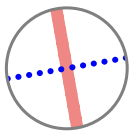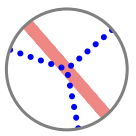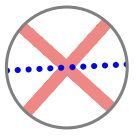시간 제한메모리 제한제출정답맞힌 사람정답 비율
2 초 (추가 시간 없음) 512 MB111100.000%

## 문제

Petya and his friends want to celebrate his birthday party! Petya has a round cake for the occassion. The only thing left is to divide it, and they will have tea.

In order to divide the cake, Petya is going to use slicers. Each slicer has a few blades: a $k$-slicer is a device which makes $k$ straight cuts from the center of the cake to its boundary, and thus divides the round cake into $k$ identical sectors.

Petya has a red $a$-slicer and a blue $b$-slicer. Fortunately, there are exactly $a + b$ friends at the party including Petya. So he decided to use each slicer once, so that the cake will be divided into exactly $a + b$ sectors.

After using the two slicers, the resulting $a + b$ sectors may have different sizes. Nevertheless, the cake should be divided as fairly as possible: the difference between the largest sector and the smallest sector should be the minimum possible.

Find out what is the minimum possible difference that Petya can achieve. Find the difference between the areas of the largest and the smallest sectors after the optimal division. Regard the area of the whole cake as $1$. Print the resulting area difference as an irreducible fraction.

## 입력

The first line of input contains two space-separated integers $a$ and $b$: the parameters of red and blue slicers ($2 \le a, b \le 100$).

## 출력

Print an irreducible fraction in the form $a$ / $b$: the difference between the areas of the largest and the smallest of the $a + b$ resulting sectors after the slicers are applied optimally.

## 예제 입력 1

2 2


## 예제 출력 1

0 / 1## 예제 입력 2

2 3


## 예제 출력 2

1 / 4## 예제 입력 3

4 2


## 예제 출력 3

1 / 8## 노트

The result of optimal use of slicers is shown to the below of the examples. The cuts made by the red $a$-slicer are shown as pale red thick lines. The cuts made by the blue $b$-slicer are shown as blue thin dotted lines.